Home Practice
For learners and parents For teachers and schools
Textbooks
Full catalogue
Pricing SupportLog in

We think you are located in United States. Is this correct?

Quadratic inequalities can be of the following forms:

\begin{align*} ax^2 + bx + c > 0 \\ ax^2 + bx + c \ge 0 \\ ax^2 + bx + c < 0 \\ ax^2 + bx + c \le 0 \end{align*}

To solve a quadratic inequality we must determine which part of the graph of a quadratic function lies above or below the $$x$$-axis. An inequality can therefore be solved graphically using a graph or algebraically using a table of signs to determine where the function is positive and negative.

## Worked example 16: Solving quadratic inequalities

Solve for $$x$$: $${x}^{2}-5x+6\ge 0$$

$(x-3)(x-2) \geq 0$

### Determine the critical values of $$x$$

From the factorised quadratic we see that the values for which the inequality is equal to zero are $$x=3$$ and $$x=2$$. These are called the critical values of the inequality and they are used to complete a table of signs.

### Complete a table of signs

We must determine where each factor of the inequality is positive and negative on the number line:

• to the left (in the negative direction) of the critical value
• equal to the critical value
• to the right (in the positive direction) of the critical value

In the final row of the table we determine where the inequality is positive and negative by finding the product of the factors and their respective signs.

 Critical values $$x=2$$ $$x=3$$ $$x-3$$ $$-$$ $$-$$ $$-$$ $$\text{0}$$ $$+$$ $$x-2$$ $$-$$ $$\text{0}$$ $$+$$ $$+$$ $$+$$ $$f(x)=(x-3)(x-2)$$ $$+$$ $$\text{0}$$ $$-$$ $$\text{0}$$ $$+$$

From the table we see that $$f(x)$$ is greater than or equal to zero for $$x\le 2$$ or $$x\ge 3$$.

### A rough sketch of the graph

The graph below does not form part of the answer and is included for illustration purposes only. A graph of the quadratic helps us determine the answer to the inequality. We can find the answer graphically by seeing where the graph lies above or below the $$x$$-axis.

• From the standard form, $$x^2 - 5x + 6$$, $$a>0$$ and therefore the graph is a “smile” and has a minimum turning point.
• From the factorised form, $$(x-3)(x-2)$$, we know the $$x$$-intercepts are $$(2;0)$$ and $$(3;0)$$.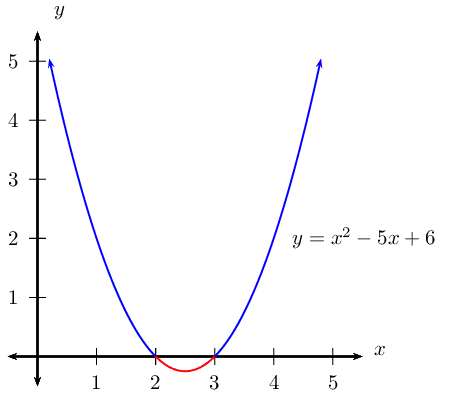The graph is above or on the $$x$$-axis for $$x \le 2$$ or $$x \ge 3$$.

### Write the final answer and represent on a number line

$x^2 - 5x + 6 \geq 0 \text{ for } x \leq 2 \text{ or } x \geq 3$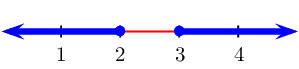temp text

## Worked example 17: Solving quadratic inequalities

Solve for $$x$$: $$4{x}^{2}-4x+1\le 0$$

\begin{align*} (2x-1)(2x-1) & \leq 0 \\ (2x-1)^2 & \leq 0 \end{align*}

### Determine the critical values of $$x$$

From the factorised quadratic we see that the value for which the inequality is equal to zero is $$x=\frac{1}{2}$$. We know that $$a^2 > 0$$ for any real number $$a, a \ne 0$$, so then $$(2x-1)^2$$ will never be negative.

### A rough sketch of the graph

The graph below does not form part of the answer and is included for illustration purposes only.

• From the standard form, $$4x^2 - 4x + 1$$, $$a > 0$$ and therefore the graph is a “smile” and has a minimum turning point.
• From the factorised form, $$(2x-1)(2x-1)$$, we know there is only one $$x$$-intercept at $$\left(\frac{1}{2};0\right)$$.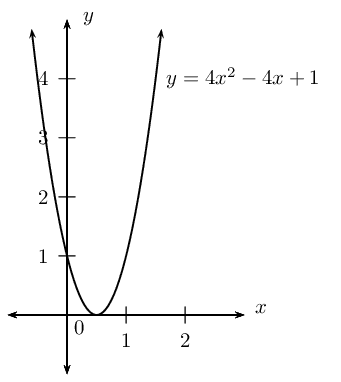Notice that no part of the graph lies below the $$x$$-axis.

### Write the final answer and represent on a number line

$4x^2 - 4x + 1 \leq 0 \text{ for } x = \frac{1}{2}$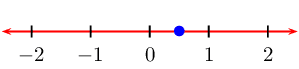temp text

## Worked example 18: Solving quadratic inequalities

Solve for $$x$$: $$-{x}^{2}-3x+5>0$$

### Examine the form of the inequality

Notice that the coefficient of the $$x^2$$ term is $$-\text{1}$$. Remember that if we multiply or divide an inequality by a negative number, then the inequality sign changes direction. So we can write the same inequality in different ways and still get the same answer, as shown below.

$-x^2-3x+5 > 0$

Multiply by $$-\text{1}$$ and change direction of the inequality sign $x^2+3x-5 < 0$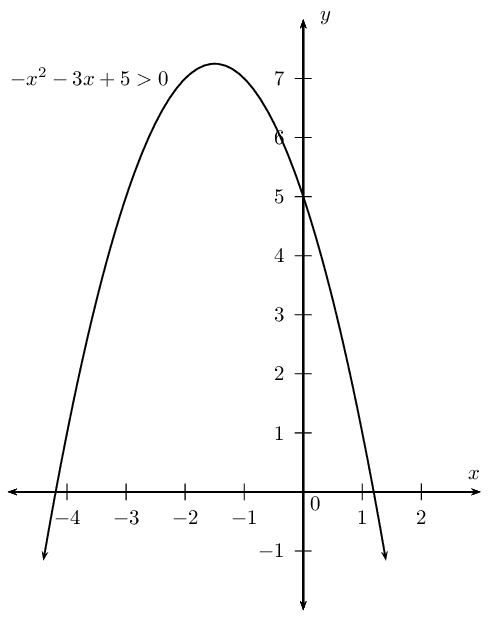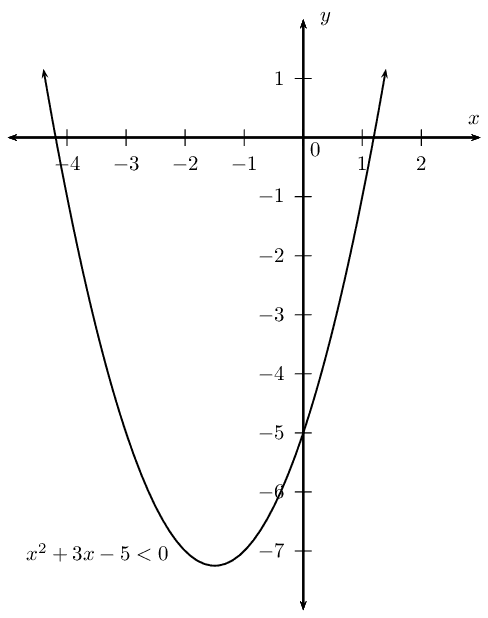From this rough sketch, we can see that both inequalities give the same solution; the values of $$x$$ that lie between the two $$x$$-intercepts.

We notice that $$-{x}^{2}-3x+5>0$$ cannot be easily factorised. So we let $$-x^2-3x+5=0$$ and use the quadratic formula to determine the roots of the equation.

\begin{align*} -x^2 - 3x + 5 &= 0 \\ x^2 + 3x - 5 &= 0 \end{align*}\begin{align*} \therefore x &= \frac{-3±\sqrt{{\left(3\right)}^{2}-4\left(1\right)\left(-5\right)}}{2\left(1\right)} \\ &= \frac{-3±\sqrt{29}}{2} \\ x_1 &= \frac{-3-\sqrt{29}}{2} \approx -\text{4,2} \\ x_2 &= \frac{-3+\sqrt{29}}{2} \approx \text{1,2} \end{align*}

Therefore we can write, correct to one decimal place, \begin{align*} x^2+3x-5 &< 0 \\ \text{as } (x-\text{1,2})(x+\text{4,2}) &< 0 \end{align*}

### Determine the critical values of $$x$$

From the factorised quadratic we see that the critical values are $$x=\text{1,2}$$ and $$x=-\text{4,2}$$.

### Complete a table of signs

 Critical values $$x=-\text{4,2}$$ $$x=\text{1,2}$$ $$x+\text{4,2}$$ $$-$$ $$\text{0}$$ $$+$$ $$+$$ $$+$$ $$x-\text{1,2}$$ $$-$$ $$-$$ $$-$$ $$\text{0}$$ $$+$$ $$f(x)=(x+\text{4,2})(x-\text{1,2})$$ $$+$$ $$\text{0}$$ $$-$$ $$\text{0}$$ $$+$$

From the table we see that the function is negative for $$-\text{4,2} < x < \text{1,2}$$.

### A sketch of the graph

• From the standard form, $$x^2+3x-5$$, $$a > 0$$ and therefore the graph is a “smile” and has a minimum turning point.
• From the factorised form, $$(x-\text{1,2})(x+\text{4,2})$$, we know the $$x$$-intercepts are $$(-\text{4,2};0)$$ and $$(\text{1,2};0)$$.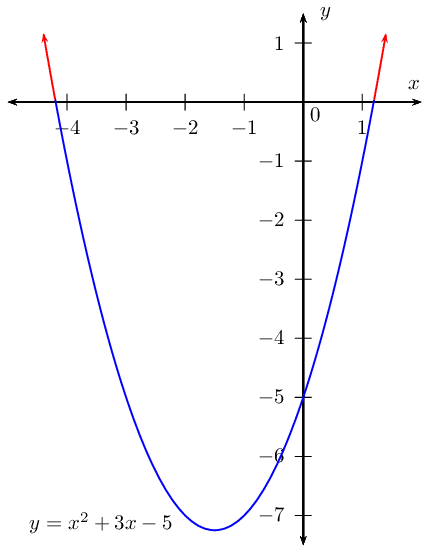From the graph we see that the function lies below the $$x$$-axis between $$-\text{4,2}$$ and $$\text{1,2}$$.

### Write the final answer and represent on a number line

$x^2 + 3x - 5 < 0 \text{ for } -\text{4,2} < x < \text{1,2}$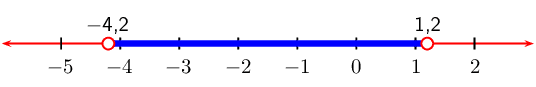Important: When working with an inequality in which the variable is in the denominator, a different approach is needed. Always remember to check for restrictions.

## Worked example 19: Solving quadratic inequalities with fractions

Solve for $$x$$:

1. $$\dfrac{2}{x+3} = \dfrac{1}{x-3}$$, $$x \ne \pm 3$$

2. $$\dfrac{2}{x+3} \le \dfrac{1}{x-3}$$, $$x \ne \pm 3$$

### Solving the equation

To solve this equation we multiply both sides of the equation by $$(x+3)(x-3)$$ and simplfy: \begin{align*} \frac{2}{x+3} \times (x+3)(x-3) &= \frac{1}{x-3} \times (x+3)(x-3)\\ 2(x-3) &= x+3\\ 2x-6 &= x+3\\ x &= 9 \end{align*}

### Solving the inequality

It is very important to recognise that we cannot use the same method as above to solve the inequality. If we multiply or divide an inequality by a negative number, then the inequality sign changes direction. We must rather simplify the inequality to have a lowest common denominator and use a table of signs to determine the values that satisfy the inequality.

### Subtract $$\dfrac{1}{x-3}$$ from both sides of the inequality

$\frac{2}{x+3} - \frac{1}{x-3} \le 0$

### Determine the lowest common denominator and simplify the fraction

\begin{align*} \frac{2(x-3) - (x+3)}{(x+3)(x-3)} &\le 0 \\ \frac{x-9}{(x+3)(x-3)} &\le 0 \end{align*}

Keep the denominator because it affects the final answer.

### Determine the critical values of $$x$$

From the factorised inequality we see that the critical values are $$x=-3$$, $$x=3$$ and $$x=9$$.

### Complete a table of signs

 Critical values $$x=-3$$ $$x=3$$ $$x=9$$ $$x+3$$ $$-$$ undef $$+$$ $$+$$ $$+$$ $$+$$ $$+$$ $$x-3$$ $$-$$ $$-$$ $$-$$ undef $$+$$ $$+$$ $$+$$ $$x-9$$ $$-$$ $$-$$ $$-$$ $$-$$ $$-$$ $$\text{0}$$ $$+$$ $$f(x)=\dfrac{x-9}{(x+3)(x-3)}$$ $$-$$ undef $$+$$ undef $$-$$ $$\text{0}$$ $$+$$

From the table we see that the function is less than or equal to zero for $$x < -3$$ or $$3 < x \leq 9$$. We do not include $$x = -3$$ or $$x = 3$$ in the solution because of the restrictions on the denominator.

### Write the final answer and represent on a number line

$x < -3 \quad\text{or}\quad 3 < x \le 9$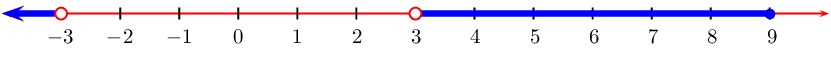Textbook Exercise 2.8

Solve the following inequalities and show each answer on a number line:

$${x}^{2}-x<12$$

\begin{align*} x^{2} - x - 12 & < 0 \\ (x - 4)(x + 3) & < 0 \end{align*}
 Critical values $$x=-3$$ $$x=4$$ $$x - 4$$ $$-$$ $$-$$ $$-$$ $$\text{0}$$ $$+$$ $$x + 3$$ $$-$$ $$\text{0}$$ $$+$$ $$+$$ $$+$$ $$f(x)= (x - 4)(x + 3)$$ $$+$$ $$\text{0}$$ $$-$$ $$\text{0}$$ $$+$$

From the table we see that $$f(x)$$ is less than $$\text{0}$$ when $$-3 < x < 4$$

We represent this on a number line: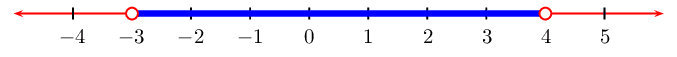$$3{x}^{2}>-x+4$$

\begin{align*} 3x^{2} + x - 4 & > 0 \\ (3x + 4)(x - 1) & > 0 \end{align*}
 Critical values $$x=-\frac{4}{3}$$ $$x=1$$ $$x - 1$$ $$-$$ $$-$$ $$-$$ $$\text{0}$$ $$+$$ $$3x + 4$$ $$-$$ $$\text{0}$$ $$+$$ $$+$$ $$+$$ $$f(x)= (3x + 4)(x - 1)$$ $$+$$ $$\text{0}$$ $$-$$ $$\text{0}$$ $$+$$

From the table we see that $$f(x)$$ is greater than $$\text{0}$$ when $$x < -\frac{4}{3}$$ or when $$x > 1$$

We represent this on a number line: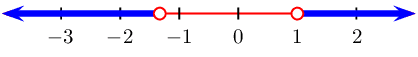$${y}^{2}<-y-2$$

$y^{2} + y + 2 < 0$

There are no real solutions.

The graph lies above the $$x$$-axis and does not cut the $$x$$-axis so the function is never negative. There are no values of $$y$$ that will solve this inequality.

$$(3-t)(1+t) > 0$$

$(3 - t)(1 + t) > 0$
 Critical values $$t = -1$$ $$t = 3$$ $$3 - t$$ $$+$$ $$+$$ $$+$$ $$\text{0}$$ $$-$$ $$1 + t$$ $$-$$ $$\text{0}$$ $$+$$ $$+$$ $$+$$ $$f(x)= (3 - t)(1 + t)$$ $$-$$ $$\text{0}$$ $$+$$ $$\text{0}$$ $$-$$

From the table we see that $$f(x)$$ is greater than $$\text{0}$$ when $$-1 < t < 3$$

We represent this on a number line: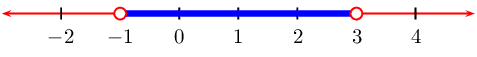$${s}^{2}-4s>-6$$

$s^{2} - 4s + 6 > 0 \\$

Use the quadratic formula to find critical values:

\begin{align*} s & = \frac{-(-4) \pm \sqrt{(-4)^2 - 4(1)(6)}}{2(1)} \\ & = \frac{4 \pm \sqrt{16- 24}}{2 } \\ & = \frac{4 \pm \sqrt{-8}}{2} \end{align*}

Therefore there are no real roots and the graph does not cut the $$x$$-axis. The graph lies above the $$x$$-axis and so this inequality is true for all real values of $$s$$.

$$0 \ge 7{x}^{2}-x+8$$

Use the quadratic formula to find critical values:

\begin{align*} x & = \frac{-(-1) \pm \sqrt{(-1)^2 - 4(7)(8)}}{2(7)} \\ & = \frac{1 \pm \sqrt{1- 224}}{14} \\ & = \frac{1 \pm \sqrt{-223}}{14} \end{align*}

Therefore there are no real roots and the graph does not cut the $$x$$-axis. The graph lies above the $$x$$-axis and so this inequality is true for all real values of $$x$$.

$$x \geq -4x^2$$

\begin{align*} x & \geq -4x^2 \\ 4x^2 + x & \geq 0 \\ x(4x + 1) & \geq 0 \end{align*}
 Critical values $$x = -\frac{1}{4}$$ $$x = 0$$ $$x$$ $$-$$ $$-$$ $$-$$ $$\text{0}$$ $$+$$ $$4x + 1$$ $$-$$ $$\text{0}$$ $$+$$ $$+$$ $$+$$ $$f(x)= x(4x + 1)$$ $$+$$ $$\text{0}$$ $$-$$ $$\text{0}$$ $$+$$

From the table we see that $$f(x)$$ is greater than $$\text{0}$$ when $$x \leq -\frac{1}{4}$$ or $$x \geq 0$$.

We can represent this on a number line: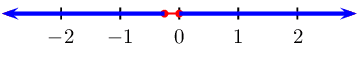$$2{x}^{2}+x+6\le 0$$

$2x^{2} + x + 6 \le 0$

There are no real roots and the graph does not cut the $$x$$-axis. The graph lies above the $$x$$-axis and so this inequality is never true.

$$\dfrac{x}{x-3}<2$$, $$x\ne 3$$

We first solve the equation:

\begin{align*} \dfrac{x}{x-3} & < 2 \\ \dfrac{x}{x-3} - 2 & < 0 \\ \dfrac{x - 2(x-3)}{x-3}& < 0 \\ \dfrac{x - 2x + 6}{x-3}& < 0 \\ \dfrac{-x + 6}{x-3}& < 0 \\ \dfrac{-(x - 6)}{x-3}& < 0 \\ \dfrac{x - 6}{x-3}& > 0 \\ x & = 6 \end{align*}
 Critical values $$x = 3$$ $$x = 6$$ $$x - 3$$ $$-$$ undef $$+$$ $$+$$ $$+$$ $$x - 6$$ $$-$$ $$-$$ $$-$$ $$\text{0}$$ $$+$$ $$f(x)= x - 6$$ $$+$$ undef $$-$$ $$\text{0}$$ $$+$$

From the table we see that $$f(x) > 0$$ when $$x < 3 \text{ or } x > 6$$ with $$x \ne 3$$.

We can represent this on a number line: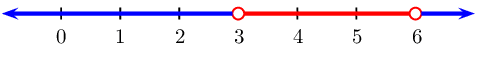$$\dfrac{x^2 + 4}{x - 7} \geq 0$$, $$x \ne 7$$

We first simplify the fraction:

$\dfrac{(x + 2)(x - 2)}{x - 7} \geq 0$
 Critical values $$x = -2$$ $$x = 2$$ $$x = 7$$ $$x + 2$$ $$-$$ $$\text{0}$$ $$+$$ $$+$$ $$+$$ $$+$$ $$+$$ $$x - 2$$ $$-$$ $$-$$ $$-$$ $$\text{0}$$ $$+$$ $$+$$ $$+$$ $$x - 7$$ $$-$$ $$-$$ $$-$$ $$-$$ $$-$$ undef $$+$$ $$f(x)= \dfrac{(x + 2)(x - 2)}{x - 7}$$ $$-$$ $$\text{0}$$ $$+$$ $$\text{0}$$ $$-$$ undef $$+$$

From the table we see that $$f(x)$$ is greater than $$\text{0}$$ when $$-2 \leq x \leq 2$$ and $$x > 7$$ with $$x \ne 7$$.

We can represent this on a number line: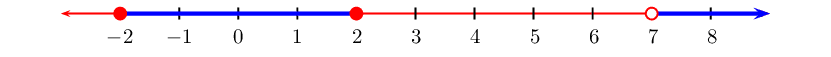$$\dfrac{x + 2}{x} - 1 \geq 0$$, $$x \ne 0$$

We first simplify the equation:

\begin{align*} \frac{x + 2}{x} - 1 & \ge 0 \\ \frac{x + 2 - x}{x}& \ge 0 \\ \frac{2}{x} & \ge 0 \\ \text{Therefore } x & > 0 \end{align*}

The solution is $$x > 0$$ with $$x \ne 0$$.

We can represent this on a number line: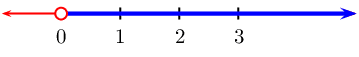Draw a sketch of the following inequalities and solve for $$x$$:

$$2x^2-18 > 0$$

We draw the parabola: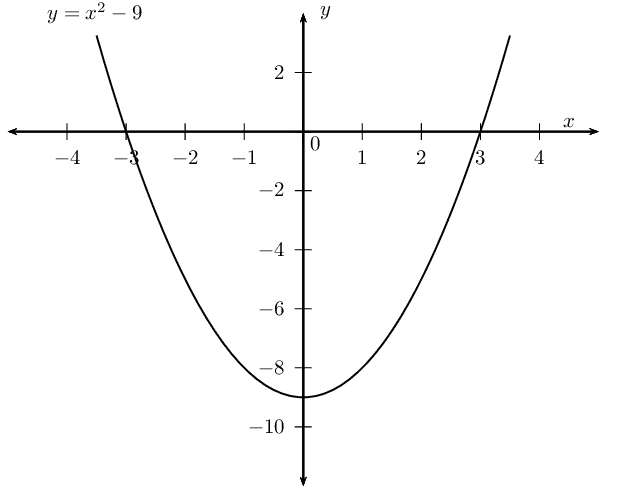And now we note that we are looking for the parts of the parabola that are greater than $$\text{0}$$. These parts are marked in red below: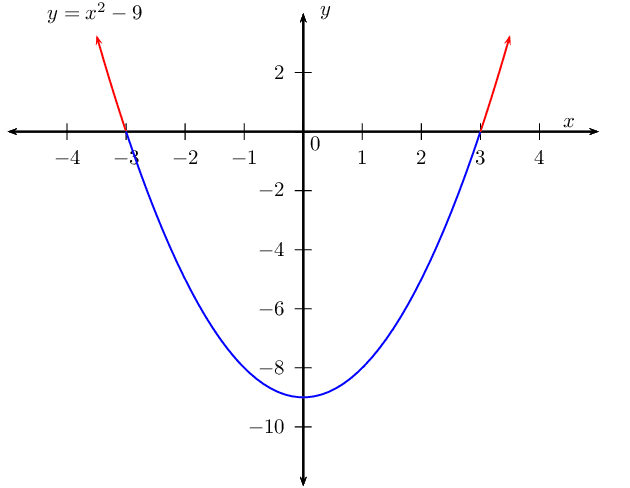This gives a final answer of $$x < -3$$ or $$x > 3$$.

$$5 - x^2 \leq 0$$

We first simplify the equation:

\begin{align*} 5 - x^2 & \leq 0 \\ - (x^2 - 5) & \leq 0 \\ x^2 - 5 & \geq 0 \\ \text{Therefore } x & > 0 \end{align*}

We draw the parabola: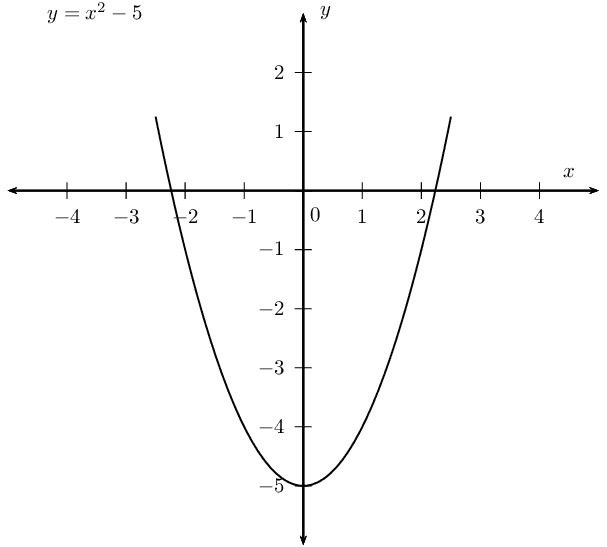And now we note that we are looking for the parts of the parabola that are less than or equal to $$\text{0}$$. These parts are marked in red below: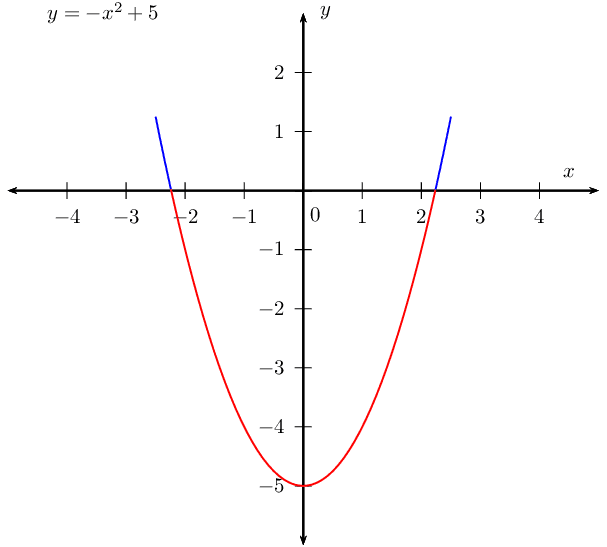This gives a final answer of $$-\sqrt{5} \leq x \leq \sqrt{5}$$.

$$x^2 < 0$$

We draw the parabola: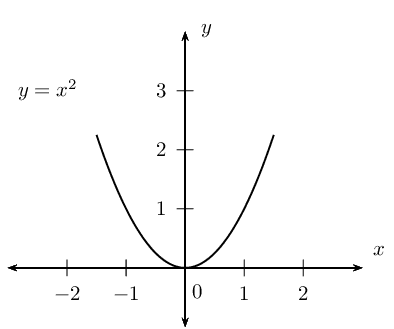We are looking for the parts of the parabola that are less than $$\text{0}$$. The parabola does not go below the $$x$$-axis and so is therefore never negative. There is no solution to this inequality.

$$0 \ge 6x^2$$

We draw the parabola: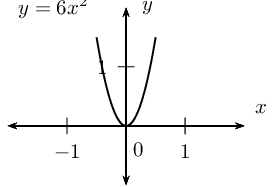We see that the parabola never touches the $$x$$-axis and is always positive. Therefore the inequality is true for all real values of $$x$$.